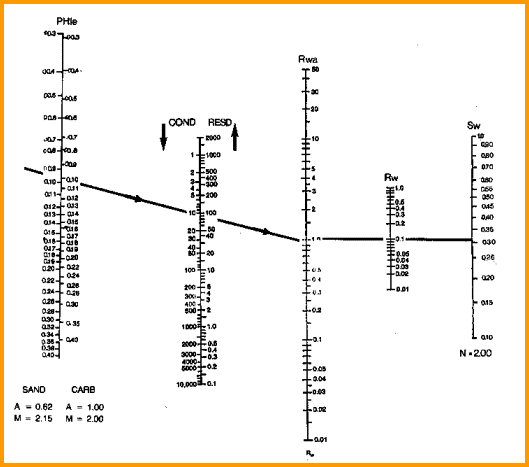ARCHIE WATER Saturation
This method is the old standard of the industry, but is now restricted to clean sands and carbonates. It requires a good value for water resistivity. All shale corrected saturation models revert to this equation when Vsh = 0, so shale corrected models are usually run when using computer-aided log analysis software.

The exponents A and M should be calibrated to the particular zone being analyzed.Water Saturation from the Archie Method
1: IF PHIe <= 0.0
2: OR IF Vsh >= 0.9
3: THEN Swa = 1.0
4: OTHERWISE Swa = (A * RW@FT / (PHIe ^ M) / RESD) ^ (1 / N)

Where:
A = tortuosity exponent (unitless)
M = cementation exponent (unitless)
N = saturation exponent (unitless)
PHIe = effective porosity (fractional)
RESD = resistivity of zone (ohm-m)
RW@FT = water resistivity at formation temperature (ohm-m)
Swa = water saturation from Archie method (fractional)

Swa2 - Water Saturation from Apparent Water Resistivity
The Archie formula can be rewritten as:
5: IF PHIe <= 0.0
6: OR IF Vsh >= 0.9
7: THEN Swa = 1.0
8: OTHERWISE Rwa = (PHIe ^ M) * RESD / A
9: AND Swa = (RW@FT / Rwa) ^ (1/N)

Where:
A = tortuosity exponent (unitless)
M = cementation exponent (unitless)
N = saturation exponent (unitless)
PHIe = effective porosity (fractional)
RESD = resistivity of zone (ohm-m)
Rwa = apparent water resistivity (ohm-m)
RW@FT = water resistivity at formation temperature (ohm-m)
Swa = water saturation from Archie method (fractional)COMMENTS:
This is a convenient form if using the Rwa values as guides to finding an appropriate RW@FT value to use in the saturation equation. This equation is not shale corrected, so it should not be used in shaly zones.

References:
1.  Electrical Resistivity Log as an Aid in Determining Some Reservoir Characteristics
G.E. Archie, Journal of Petroleum Technology, 1941

2.
Resistivity of Brine Saturated Sands in Relation of Pore Geometry
W.O. Winsauer, H.M. Shearin, P.H. Masson, and M. Williams. AIME, 1952

A quick look version of this formula sets A = 1.0, M = 2.0 and N = 2.0. Thus:
10: Swa = (RW@FT / (PHIe ^ 2) / RESD) ^ 0.5

This formula can be calculated by mental arithmetic or on a scratch pad when needed, and is accurate enough for quick look work.

The graphical solution to the Archie formula is provided below.Nomograph to solve Archie equationRECOMMENDED PARAMETERS:
for carbonates A = 1.00  M = 2.00  N = 2.00  (Archie Equation as first published)
for sandstone  A = 0.62  M = 2.15  N = 2.00  (Humble Equation)
A = 0.81  M = 2.00  N = 2.00  (Tixier Equation - simplified version of Humble Equation)
NOTE: N is often lower than 2.0

For quick analysis use carbonate values. Values for local situations should be developed from special core data. Results will always be better if good local data is used instead of traditional values, such as those given above.

Asquith (1980 page 67) quoted other authors, giving values for A and M, with N = 2.0, showing the wide range of possible values:

Average sands              A = 1.45  M = 1.54
Shaly sands                  A = 1.65  M = 1.33
Calcareous sands         A = 1.45  M = 1.70
Carbonates                   A = 0.85  M = 2.14
Pliocene sands S.Cal.  A = 2.45  M = 1.08
Miocene LA/TX             A = 1.97  M = 1.29
Clean granular             A = 1.00  M = 2.05 - PHIeNUMERICAL EXAMPLE: 1. Using data from Sands A, B, C and D as before: Sand A Sand B Sand C Sand D RESD 20 40 1.2 1.0 PHIe 0.33 0.23 0.30 0.11 A 0.62 0.62 0.62 0.62 M 2.15 2.15 2.15 2.15 Rwa 2.97 2.73 0.145 0.014 RW @ FT 0.9 0.9 0.036 0.015 N 2.0 2.0 2.0 2.0 Swa 0.55 0.57 0.50 1.03

Sample:
Swa = (0.62 * 0.9 / (0.33 ^ 2.15) / 20) ^ (1 / 2.0) = 0.55

Note that the value for Sand D (shaly sand) is inaccurate and requires additional work, because this method is poorly suited to shaly sands.

Page Views ---- Since 01 Jan 2015
Copyright 2023 by Accessible Petrophysics Ltd.
CPH Logo, "CPH", "CPH Gold Member", "CPH Platinum Member", "Crain's Rules", "Meta/Log", "Computer-Ready-Math", "Petro/Fusion Scripts" are Trademarks of the Author